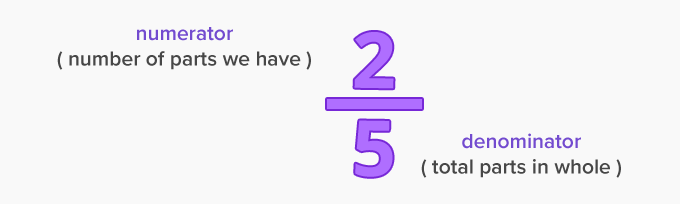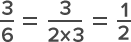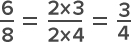# Simplest Form - Definition with Examples

The Complete K-5 Math Learning Program Built for Your Child

• 40 Million Kids

Loved by kids and parent worldwide

• 50,000 Schools

Trusted by teachers across schools

• Comprehensive Curriculum

Aligned to Common Core

## What is Simplest Form?

A fraction is not a whole number but a number in between the whole numbers. It is a part of a whole. Also, a fraction has two parts – a numerator and a denominatorWhen the numerator and the denominator can no longer be reduced to any smaller number separately, we get the fraction in its simplest form.

For example,

36 can be simplified as :In the given example, we obtain the simplified form by dividing the numerator and denominator by 3, the greatest number that divides both the numbers exactly (reducing them into another whole number).

Thus, finding the simplest form of a fraction means reducing the top and bottom of the fraction to the smallest whole number possible.

 Fun Facts The simplest form is the smallest possible equivalent fraction of the number.

Steps for finding the simplest form:

• Search for common factors in the numerator and denominator.

• Check whether one of the numbers in the fraction is a prime number.

(Revise your knowledge: A prime number is a number which has only two factors 1 and itself.  )

• Divide by a fraction equivalent to 1. (Multiplying would make the original fraction larger, which is not what we want when simplifying.)  For example, 231010 , or 55 are all equivalent to 1.

For example,

Jenny has a chocolate cake which she has cut into 6 pieces. She shares this equally with 7 other friends, which makes a total of 8 persons.

So that leaves Jenny with a fraction 6. Can this be any simpler?

None of the numbers (the top and bottom of this fraction) is a prime number.

Let's look at the common factors :

6 → 2,3,6

8 → 2,4,8

So 2 is a common factor of the numerator and denominator.So the simplest form is 3

Thus each set of 4 people will get to share 3 pieces of cake.

Won Numerous Awards & Honors